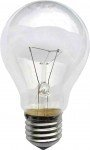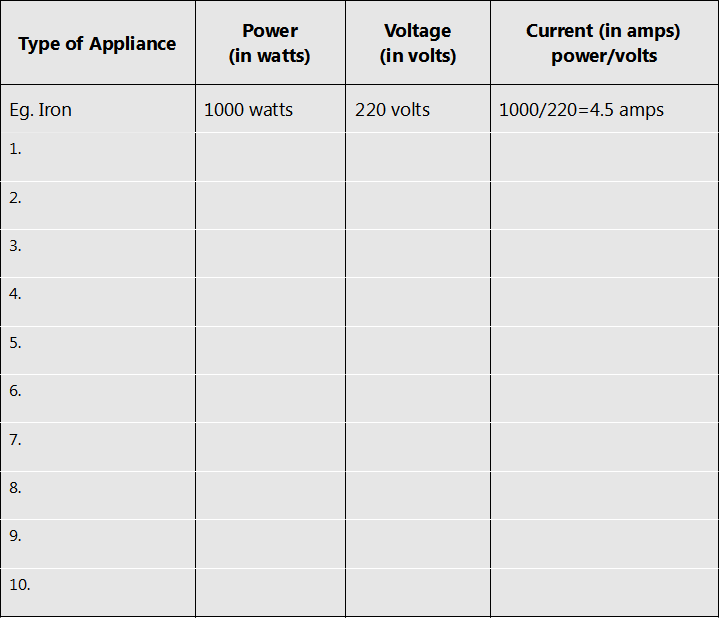Home Fundamentals What is Electrical Power?

# What is Electrical Power?

What is electrical power? What is the formula for electrical power? What is watts, volts and amps? This article introduces and illustrates these electrical basics and in particular the power formula. As this is not a text book, the formula is not proved or derived.  The power formula is simply introduced and used to show some of the important principles needed in understanding and fault finding electrical or electronic things.

If you are a self-confessed electrical illiterate, then you are encouraged to read through this so that you are familiar enough to come back and use this as a future reference.

Those of you who have mental blocks when you see anything technical, or see formulas, tables and graphs, be assured there are not too many here, only the minimum necessary to illustrate the fundamental principles of electrical power are used.

## What is Power?

Let’s start by asking a question: Which of these vehicles is more powerful?Certainly a tractor has a lot pushing or pulling force, or grunt, so can obviously be deemed powerful, but it can’t go very fast. A racing car, on the other hand, goes extremely fast and is called powerful accordingly, but it can’t pull heavy loads. A sports car can’t pull as much as a tractor, and can’t go as fast as a racing car, but is none the less, very powerful.

So it boils down to our definition of power. We could simply say the power of the vehicle is the combination of its ability to push (or pull) and its speed. That is, if we had a figure for the pushing (or pulling) force of a certain vehicle (we’ll call this “grunt”), and we knew its speed, then we could derive the formula:

##### Power of Vehicle  =  Grunt x Speed

If the following substitutions are made to get “electrical” power:

##### Grunt = Current                           Speed = Voltage

then the formula for electrical power looks like this:

##### Electrical Power = Current x Voltage

This simple formula is one of the most important ones you will need to know for electrical work.

Knowing the common symbols and units of measurement for these characteristics is useful, and can make you sound like you really know your stuff.

NameSymbolUnits of Measurement
PowerPwatts (W)
CurrentIamperes or amps (A)
VoltageVvolts (V)

*Text books often use “E” as the symbol for voltage. This is technically correct as the proper name for voltage is Electro-Motive Force, or EMF, which the “E” symbolises. However for ease of comprehension “V” will be used, which is easier to associate with the common understanding of Voltage

That is, the formula can be written as:

##### P = I x V

This means that for an appliance (such as a light), which draws 1.5 amps at 12 volts, the power used by the light is calculated with this formula, therefore:

Power of Light = 1.5 amps x 12 volts = 18 Watts

If you know the wattage (power) of a 12 volt light is 18 watts, then obviously the formula can be changed around to calculate the current drawn by the light. That is:

Current = Power divided by voltage

or

#### What’s it all mean?

OK, enough theory for the moment. What does all this mean in practice? Try and follow these examples:

Example 1: You have a 60 watt, 240 volt light bulb. How much current does itdraw?

I = P/V therefore Current = 60/240 = 1/4 amp.

Example 2: You have another 60 watt light bulb, but this is from your car, so it is rated at 12 volts. How much current dos this one draw?I = P/V therefore Current = 60/12 = 5 amps.

This doesn’t mean that one light is more powerful than the other, as both draw 60 watts of electrical power. However, it shows the relationship between voltage, current and electrical power. That is, for a given power (say 60 watts), if the voltage is low (12 volts), the current must be high (5 amps), and if the voltage is high (240 volts), the current will be low (¼ amp). A bit like our illustration of a tractor and a racing car: if you don’t have speed (voltage) you will need grunt (current) to get up a hill (e.g. a tractor). Similarly, if you don’t have grunt (current), you will need speed (voltage) to get up the same hill (e.g. a fast car). Please note, that like a tractor or car, don’t use the light bulb designed for one job to try to do the job of the other. In other words, don’t plug your 12 volt light into 240 volts.

##### Practical Points to note

1) An appliance only draws as much electrical power as it requires, you can’t push more electrical power into something than what it needs.

Example: If a light is rated at 60 watts, and you have a 1000 watt generator, then that is fine, but the light will only draw its 60 watts.

2) An appliance only draws as much current as it requires, you can’t make it take more Amps than it needs.

Example: If an electronic device is rated at 6 volts, 0.3 amps, and the power supply (battery eliminator or “plug pack” supply) is rated at 6 volts, 0.5 amps, then that too is fine, but the device will only draw the 0.3 amps it requires.

3) An appliance will generally work on slightly higher or slightly lower voltage than its rating. Normally, you should try to supply the correct voltage to all appliances.

4) If an appliance is rated at 1500 watts, it needs 1500 watts (at its specified voltage) of electrical power to work properly.

Example: If you have an electric drill rated at 1500 watts, and you try to run it from a 1000 watt generator, then it won’t work properly, and could even damage the drill and/or the generator.

5) The power rating of an appliance refers to either the power it puts out or the power it takes in.

Example 1: A generator rated at 1000 watts means it is capable of supplying up to 1000 watts of electrical power, at the specified voltage (like 220 volts).

Example 2: A light bulb rated at 60 watts, means it takes in 60 watts of electrical power to operate properly.

Example 3: A 300 watt inverter (say 12 Volts to 110 Volt) indicates it puts out 300 watts of electrical power at 110 volts, which means it will draw more than the 300 watts (due to efficiency losses) from the 12 volt battery (Note: 300 watts at 12 volts is 25 amps!)

6) The power rating of a light suggests the electrical power drawn, not the amount of light it puts out. A 20-watt fluorescent tube can put out more light than a 45-watt light bulb, because the fluorescent is more efficient at converting the electrical power to lighting power than the light bulb.

7) Transformers and motors are often measured in VA (Volts-Amps) or kVA (kilo Volt-Amps, i.e. 1000 volt-amps). For most purposes this rating can be equated to electrical power in watts, although in the strict technical sense there are differences (due to the current being out of phase with the voltage in an inductive circuit).

8) The electrical power required for an appliance is measured in watts or VA. This is normally written underneath or on the back of the appliance. To calculate the current drawn by an appliance, divide the figure for watts, by the voltage.

When you don’t have a calculator handy, “ball park” figures can be used. For ease of calculation, try using the following figures:

For 240 volts use 250 volts e.g. 1000/250 = 4 amps

For 220 volts use 200 volts e.g. 1000/200 = 5 amps

For 110 volts use 100 volts e.g. 1000/100 = 10 amps

Exercise: Inspect 10 different appliances around your house or office and determine how much current they each draw. Use the following table or one like it.9) To see how many amps you are drawing, simply add the amps of each appliance you are using together.

Example: Your iron draws 4 amps, your room heater draws 10 amps, together they are drawing 14 amps.

10) A4 way power board normally has a switch that cuts out when 10 amps or more is going through it. So if you have your heater and iron plugged into the one board, it will cut out and neither will work.

A simple calculator for these formula is available here.

In the next article we will see how simple the dreaded Ohms law really is…